# Electric Charge and Coulombs Law Fundamental Charge The

• Slides: 18Electric Charge and Coulomb’s Law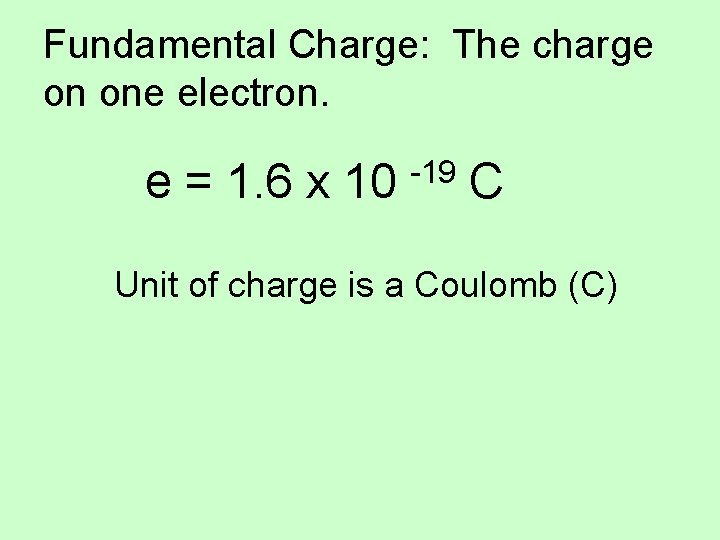Fundamental Charge: The charge on one electron. e = 1. 6 x 10 -19 C Unit of charge is a Coulomb (C)Two types of charge: Positive Charge: A shortage of electrons. Negative Charge: An excess of electrons. Conservation of charge – The net charge of a closed system remains constant.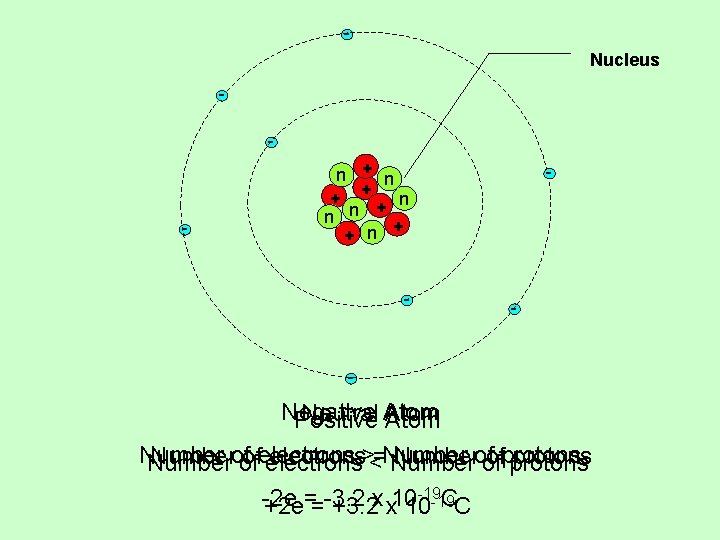Nucleus - - n + + n - - Negative Neutral Atom Positive Atom Numberof ofelectrons><=Numberof ofprotons Number of electrons Number of protons -19 -2 e = -3. 2 x 10 CC +2 e = +3. 2 x 10 -19Electric Forces Like Charges - Repel F + + Unlike Charges - Attract - F F + FCoulomb’s Law – Gives the electric force between two point charges. Inverse Square Law k = Coulomb’s Constant = 9. 0 x 109 Nm 2/C 2 q 1 = charge on mass 1 q 2 = charge on mass 2 r = the distance between the two charges The electric force is much stronger than the gravitational force.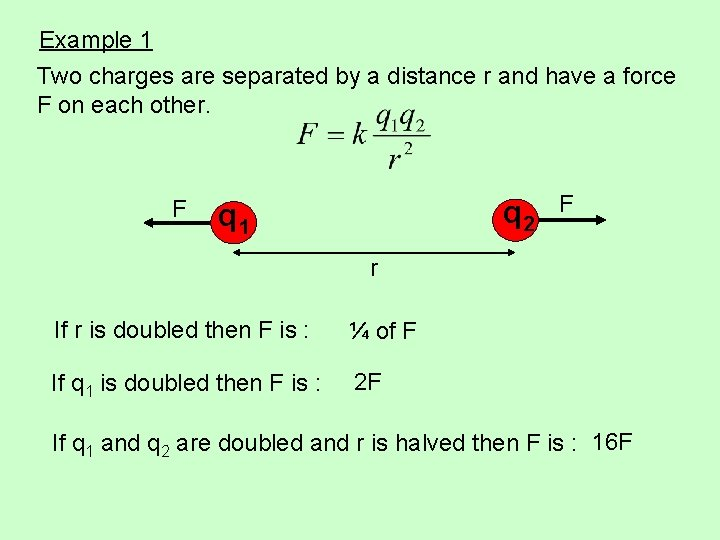Example 1 Two charges are separated by a distance r and have a force F on each other. F q 2 q 1 F r If r is doubled then F is : ¼ of F If q 1 is doubled then F is : 2 F If q 1 and q 2 are doubled and r is halved then F is : 16 F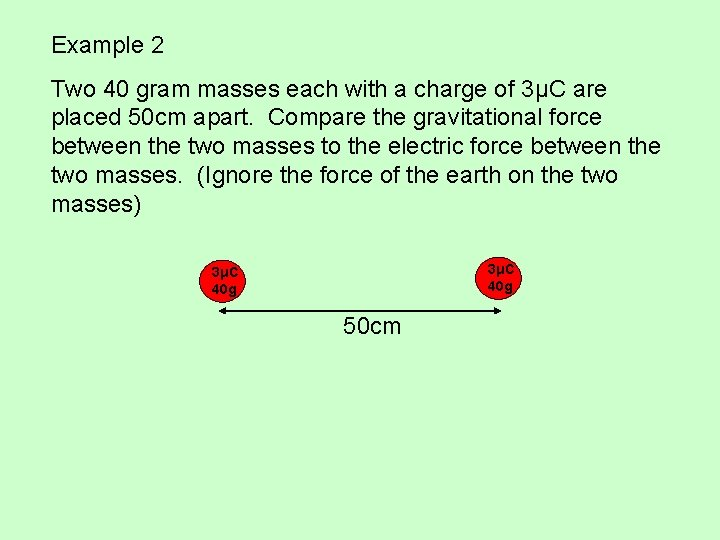Example 2 Two 40 gram masses each with a charge of 3μC are placed 50 cm apart. Compare the gravitational force between the two masses to the electric force between the two masses. (Ignore the force of the earth on the two masses) 3μC 40 g 50 cm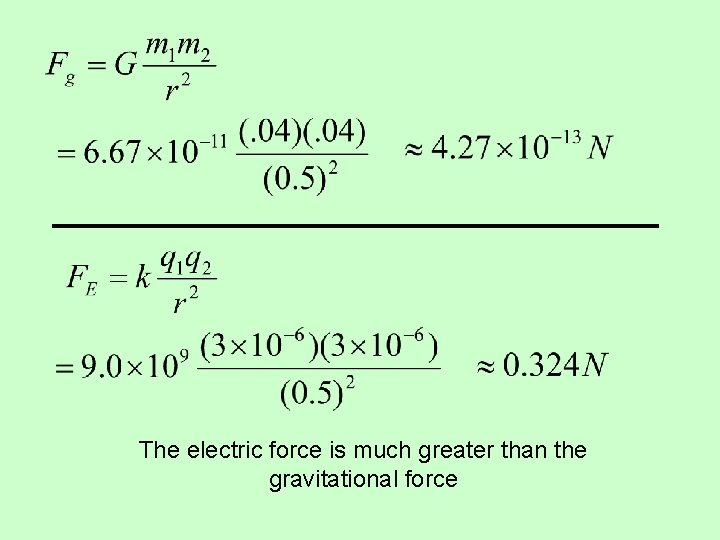The electric force is much greater than the gravitational force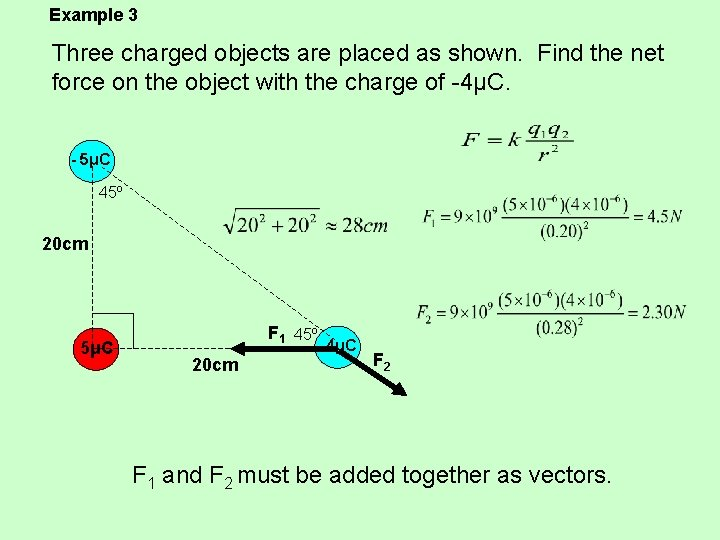Example 3 Three charged objects are placed as shown. Find the net force on the object with the charge of -4μC. - 5μC 45º 20 cm 5μC F 1 45º - 4μC 20 cm F 2 F 1 and F 2 must be added together as vectors.F 1 2. 3 cos 45≈1. 6 45º F 2 2. 3 sin 45≈1. 6 - 2. 9 29º θ 3. 31 F 1 = < - 4. 5 , 0. 0 > + F 2 = < 1. 6 , - 1. 6 > Fnet = < - 2. 9 , - 1. 6 > 3. 31 N at 209º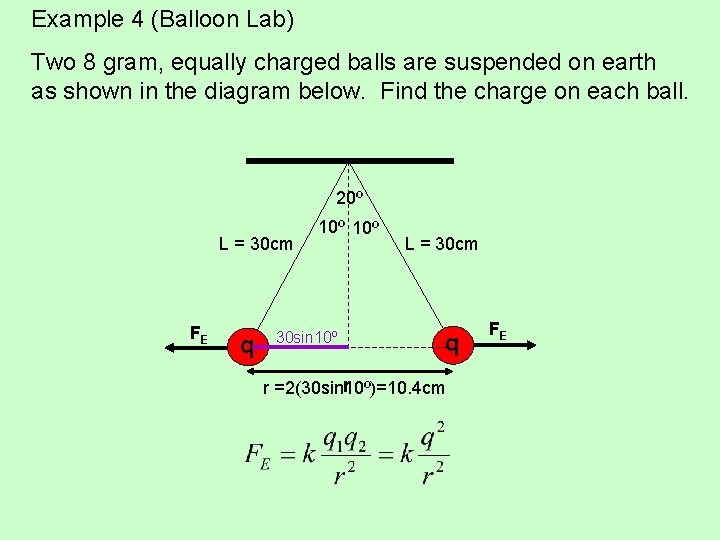Example 4 (Balloon Lab) Two 8 gram, equally charged balls are suspended on earth as shown in the diagram below. Find the charge on each ball. 20º L = 30 cm FE q 10º 30 sin 10º L = 30 cm q r r =2(30 sin 10º)=10. 4 cm FE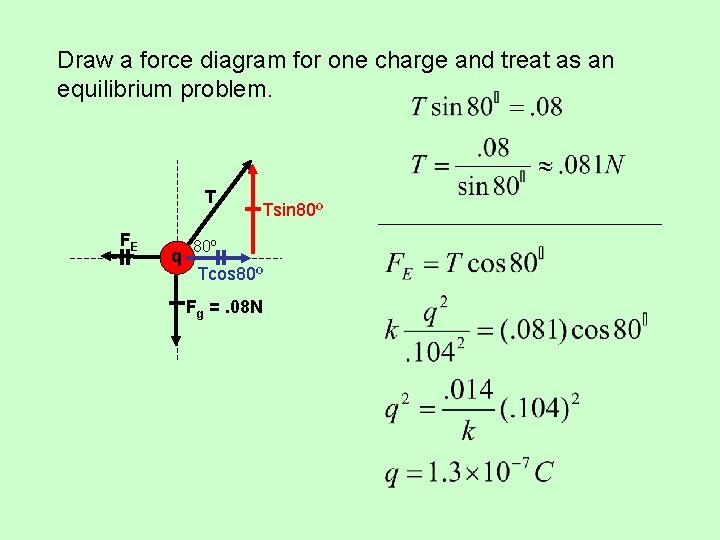Draw a force diagram for one charge and treat as an equilibrium problem. T FE q Tsin 80º Tcos 80º Fg =. 08 N